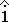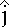+x is to the right; +y is up

Vector A has a length of 3.76 cm and is at an angle of 34.5 degrees above the positive x-direction. Vector B has a length of 4.53 cm and is at an angle of 34.1 degrees above the negative x-direction.

What is the sum (resultant) of the two vectors?

The component method of vector addition is the standard way to add vectors. If C = A + B, then:
Cx = Ax + Bx
Cy = Ay + By

Vectorx componenty component
A Ax = +3.76 cos(34.5)
Ax = +3.10 cm
Ay = +3.76 sin(34.5)
Ay = +2.13 cm
B Bx = -4.53 cos(34.1)
Bx = -3.75 cm
By = +4.53 sin(34.1)
By = +2.54 cm
C Cx = Ax + Bx
Cx = -0.65 cm
Cy = Ay + By
Cy = +4.67 cm

State the resultant like this:

C = -0.65 cm+ 4.67 cmOr, glue the two components of C together to find the magnitude and direction of C.

C2 = Cx2 + Cy2 = 0.652 + 4.672
C = 4.72 cm

tan(q) = 4.67 / 0.65

q = 82.1 degrees

So, the resultant vector has a magnitude of 4.72 cm and is 82.1 degrees above the -x direction.